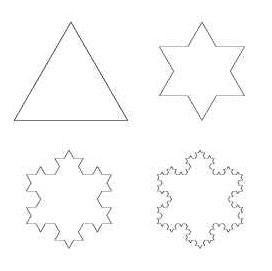Project II Template

Introduction

Few if any, know of the connections between Euler's famous equation $e^{i\pi}=-1$ and the Koch snowflake. Of course, this is just mathematical nonsense.

Describe something with a nice inline equation

(1)
\begin{align} e^{i\pi}=-1, \end{align}

which you can refer back to later (1). You can also use figuresFigure 1. The first few iterations of the Koch snowflake.

Following up with some more text.